# Calculating Infinity: Rich vs. Poor Data Representation

In a previous post, we briefly touched on the importance of rich data representation and how crucial it is to artificial intelligence systems like Infinity. But what do we mean when we say “rich representation of data”? Is there such a thing as “poor representation of data”? Let’s dive down the rabbit hole and discuss how the quality of data representation directly affects a learning algorithm.

How Machines Learn

Before we get into what constitutes rich data representation, we’ll need to first understand the basics of how machines learn. In all actuality, it’s not much different from how children begin to learn about the world they occupy. In order to teach a child the meaning of a word, we show them a visual representation of an object while repeating the word associated with that object.

During the initial training phase of a machine learning system, we feed the machine data and explain what that data represents. If our data set consists of cat photos, we’ll input that data into the machine and classify each photo as one representing a cat. If we then expand the training data to include photos of dogs, the end result should be a machine that can correctly differentiate between photos of cats and dogs without human intervention. A well-trained machine will be extremely adept at determining if a photo is of a cat or a dog, while a poorly trained one will produce results that are no better than a random coin flip.

The Decision Boundary

The overall goal for our training phase is to define a “decision boundary”. To explain what a decision boundary is, imagine that you have a graph with various points plotted where each point represents a photo of either a cat or a dog. A decision boundary is the line that separates these two distinct groups. Ideally the decision boundary is drawn so that one side contains all of the points from the cat group, and the other side contains all the points from the dog group.

It’s worth noting that not all machine leaning approaches utilize a training phase, nor do they all generate decision boundaries. Our focus here is to outline a general-purpose approach to machine learning.

Cats, Dogs, and Machines

Now that we’ve covered the basics, we can start to look at how one can present data to a machine learning system, and how the effects of different representations of the same object vastly influence the ability of a system to effectively classify information. In the end, we want to represent this data in a format that can be plotted on a graph. At first glance, it may not make sense to plot something as complex as a photo on a graph, but one can easily plot the features of a photo like the size, color, and so on.

Along with plotting the features of our data, we’re also going to tie together the ideas of training and decision boundaries. One should note that the data and assumptions we’ll be making about cats and dogs are not based on any actual measurements. The data itself is completely manufactured to help illustrate complex concepts in a comprehensible manner suitable for this blog post.

For the purpose of showing the effects of a poor data representation, the first thing we’re going to do is extract a single feature from a photo. Let’s use the brightness of the first pixel, and represent that as a single number. In this experiment, a value of 0 will represent complete darkness, and a 1 would be as bright as possible. The data that falls in-between the two values would be represented by a decimal. Now that we have a numerical scale to classify our  “brightness of the first pixel” characteristic, we can plot it on a graph.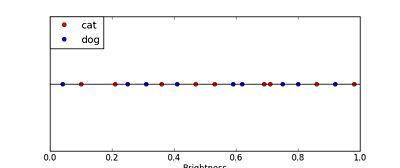In this first graph, the red circles represent the color of the first pixel in cat photos, and the blue circles represent the color of the first pixel in dog photos. As you can tell by looking at the graph, the dots are rather randomly spread out. If we were to feed this data as training data to a machine learning algorithm, it will attempt to draw a decision boundary to separate out the red (cat) and blue (dog) samples. Here is what a decision boundary would look like on this data: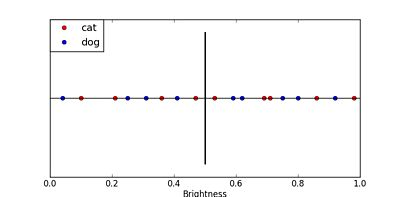Now that a decision boundary has been constructed, the machine learning algorithm can state that anything to the left of the boundary will be classified as blue, and anything to the right will be classified as red (or vice versa). However, looking at this decision boundary, one can see that both red and blue dots exist on both sides, and in mostly even numbers. In this case, the classification would be wrong about 50% of the time, which is about as poor as a machine learning system can perform.

It’s worth noting that decision boundaries are not necessarily straight lines, in fact they can be extremely complex shapes. For example, one could draw a line with numerous curves that weave in and out of the plotted points to correctly separate the blue and red dots.

The Plot Thickens

The previous example shows that our machine learning system is going to have problems trying to discern if a photo is of a cat or a dog if it relies solely on our “brightness of the first pixel” characteristic. Clearly using the brightness of the first pixel is a poor approach. Perhaps a more informative feature would make it easier to find a good decision boundary.

Another feature we could extract from a photo might be the width of the animal. We can express the width of the animal as a proportion of the total width of the photo. For example, if we can find a dog in a photo, and state that the width of the dog is 50% of the photo, then we can plot the width on a graph. Here is what a plot of samples where animal width is expressed as a percentage of the total width of the photo: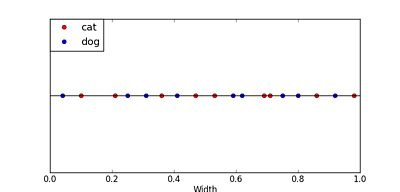While this may seem like a better feature to use than the color of the first pixel, in reality it has the same problem.

We can also apply the same feature extraction in terms of the height of the animal. Not surprisingly, the results are similar to width: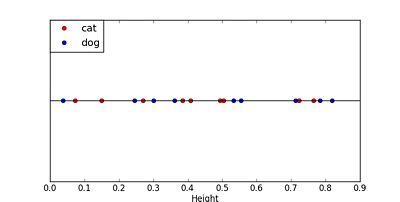It seems as if there isn’t a good, simple decision boundary that we can construct on either of these features. We then might attempt to classify our two categories by making a decision on the height and width of the subject in the photo.

Upon further examination, it quickly becomes apparent that the position of the animal in relation to the lens directly affects the both the perceived width and perceived height of an animal in proportion to the total photo size. Since we don’t have a consistent perspective for each photo, we can’t rely on width/height to provide information useful to our construction of a decision boundary.

Thinking Dimensionally

There are a few things that we can do to improve our data representation in order to generate an effective decision boundary. Typically, increasing the number of dimensions can provide a clearer separation between two groups. Increasing the number of features also helps us create a clear decision boundary.

To show the effect increasing dimensionality can have, let’s revisit the width and height examples. This time, instead of plotting a one-dimensional view of width and height separately, we’re going to plot a two-dimensional representation, where width is one axis, and height is the other. Here’s what these two features look like on a single graph: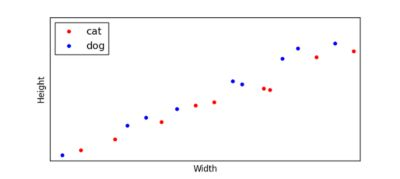Here’s what a decision boundary might look like for this data: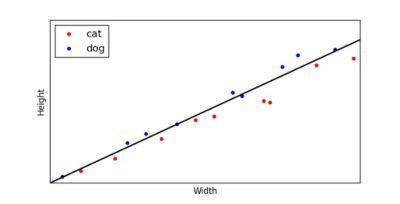When we observe this decision boundary, we can see that 5 of the blue dots (dogs) are above the line, while all of the red dots (cats) are on the other side. If we were to plot out new samples on this graph, it’s likely that most of the new samples that came from dog photos would end up on the side of the decision boundary containing blue dots, while most of samples from cats would end up on the side consisting of red dots.

For a machine learning algorithm, correctly classifying around 80% of samples would be considered a decent result. But what happens if we add more features, increasing the number of dimensions we plot? For a 3rd dimension, imagine we have a feature that represents the length of the tail in relation to the width of the animal.

Here is a three-dimensional plot of these features, where we take a similar viewpoint to the two-dimensional plot we just reviewed: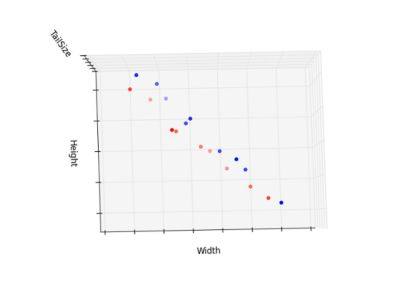And another view of the same graph from a different angle: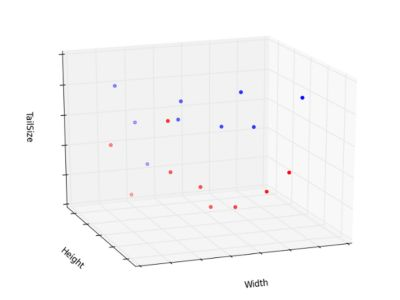In three dimensions it looks like we can create a decision boundary that splits our two groups effectively. When you compare this example to the first one, the importance of having rich data representation becomes clear.

Hopefully, this provides you with an idea of not only how machine learning systems learn from data and use that “knowledge” to classify future samples, but the importance of good data representations. Of course, machine learning systems are immensely more complicated than what’s presented here, but our aim is to give the reader a peek into the inner workings of these systems. As an example of the potential complexity of such systems, consider that Infinity uses well over one million dimensions of data to construct its decision boundaries. And no, we're not going to plot what that would look like. We'll leave that as an exercise for the reader.

- The Infinity Team